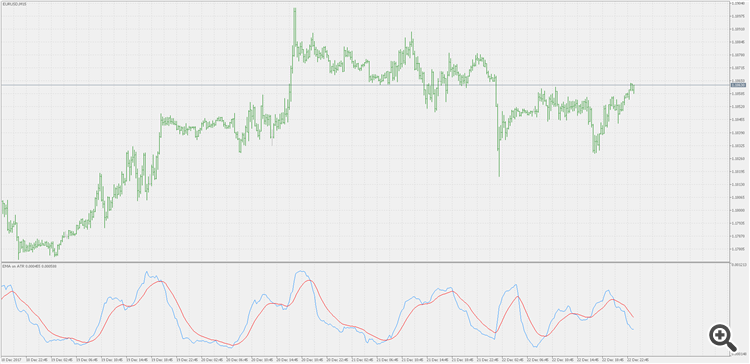# Adding EMA to ATR in MQL5 EA.

salirazataqvi:

Hi all,

I am trying to add EMA to ATR in MQL5 EA.

ATR line (Blue)

EMA line (Red)

Please tell me how to get it done in MQL5.

Thanks.

Use MovingAverages.mqh and then use ExponentialMAOnBuffer() function from that file on the buffer where you stored atr values

thanks for your reply... I used the mentioned function in my code however unable to get the desired result. the moving average is being draw in chart window not in ART window. following is my code, please tell me what am I doing wrong.

```void CalculateATR_EMA(string symbol)
{

double myPriceArray[];

int averageTrueRangeDefination = iATR(symbol, Period(), 14);

ArraySetAsSeries(myPriceArray, true);

CopyBuffer(averageTrueRangeDefination, 0, 0, 3, myPriceArray);

MqlRates priceInfo[];

ArraySetAsSeries(priceInfo, true);

int data = CopyRates(symbol, _Period, 0, 3, priceInfo);

double myMovingAverageArray[];

int movingAverageDefinatin = iMA(symbol, _Period, 14, 0, MODE_EMA, PRICE_CLOSE);

ArraySetAsSeries(myMovingAverageArray, true);

CopyBuffer(movingAverageDefinatin, 0, 0, 3, myMovingAverageArray);

ExponentialMAOnBuffer(4, 0,   0, 14, myPriceArray, myMovingAverageArray);

//double averageTrueRangeValue = NormalizeDouble(myPriceArray, 5);
}```

thanks.

salirazataqvi:

thanks for your reply... I used the mentioned function in my code however unable to get the desired result. the moving average is being draw in chart window not in ART window. following is my code, please tell me what am I doing wrong.

thanks.

Here is an "as simple as it gets" example

```#property indicator_separate_window
#property indicator_buffers 2
#property indicator_plots   2
#include <MovingAverages.mqh>

input int AtrPeriod = 14; // ATR period
input int EmaPeriod = 14; // EMA period

double atrBuffer[],emaBuffer[];

//------------------------------------------------------------------
//
//------------------------------------------------------------------
//
//
//
//
//

void OnInit()
{
SetIndexBuffer(0,atrBuffer,INDICATOR_DATA); PlotIndexSetInteger(0,PLOT_DRAW_TYPE,DRAW_LINE); PlotIndexSetInteger(0,PLOT_LINE_COLOR,clrDodgerBlue);
SetIndexBuffer(1,emaBuffer,INDICATOR_DATA); PlotIndexSetInteger(1,PLOT_DRAW_TYPE,DRAW_LINE); PlotIndexSetInteger(1,PLOT_LINE_COLOR,clrRed);
}
void OnDeinit(const int reason) { }
int OnCalculate(const int rates_total,
const int prev_calculated,
const datetime& time[],
const double& open[],
const double& high[],
const double& low[],
const double& close[],
const long& tick_volume[],
const long& volume[],
{
static int _atrHandle =  INVALID_HANDLE;
if (_atrHandle == INVALID_HANDLE)
_atrHandle  = iATR(_Symbol,_Period,14);
if (_atrHandle == INVALID_HANDLE || BarsCalculated(_atrHandle)<rates_total) return(prev_calculated);

//
//
//
//
//

int to_copy = (prev_calculated>rates_total || prev_calculated<=0) ? rates_total : rates_total-prev_calculated+1;

if(CopyBuffer(_atrHandle,0,0,to_copy,atrBuffer)<=0) return(0);
ExponentialMAOnBuffer(rates_total,prev_calculated,0,EmaPeriod,atrBuffer,emaBuffer);
return(rates_total);
}```Use that code as a sample. But trying to calculate ema on only 4 values (as you have tried) might lead to highly misleading results. Ema needs more past data (unlike some other average types) for a "stable" result. In any case, replace the value assigned to to_copy with your desired fixed number of bars, and it should work for you in an EA code as well

I have put the above provided code in custom indicator and it compiled successfully, I used it via iCustom(.......) function. the issue is that when I run the expert advisor, the expert attach the indicator again and again. Please refer to the following snapshot:

following is the block of code where I used iCustom(...) method to add this indicator:

```void OnTick()
{
int emaATRDefination = iCustom(_Symbol, _Period, "ATR_EMA");

if(emaATRDefination == INVALID_HANDLE)
Print("Handle invalid for ... error= ", GetLastError());
}
```

Moreover,  please tell me how to get buffer values out of indicator.

Thanks.

salirazataqvi:

I have put the above provided code in custom indicator and it compiled successfully, I used it via iCustom(.......) function. the issue is that when I run the expert advisor, the expert attach the indicator again and again. Please refer to the following snapshot:

following is the block of code where I used iCustom(...) method to add this indicator:

Moreover,  please tell me how to get buffer values out of indicator.

Thanks.

Happy new year ...Documentation on MQL5: Technical Indicators / iCustom
• www.mql5.com
[in]  The name of the custom indicator, with path relative to the root directory of indicators (MQL5/Indicators/). If an indicator is located in a subdirectory, for example, in MQL5/Indicators/ [in] input-parameters of a custom indicator, separated by commas. Type and order of parameters must match. If there is no parameters specified, then...

Thanks for your Sample instructions, ive tried modifying the code to include the Indicator_Color, the indicator does change its color, however, the points at which it changes seems a little off. Would you mind taking a look at it for me?

```#property indicator_chart_window
#property indicator_buffers   2
#property indicator_plots     1
#property indicator_type1     DRAW_COLOR_LINE
#property indicator_color1    clrDarkGray,clrGoldenrod,clrSteelBlue
#include  <MovingAverages.mqh>

input     int                 ma_period   =  30;
input     int                 ma_shift    =  0;
input     ENUM_MA_METHOD      ma_method   =  MODE_EMA;
input     ENUM_APPLIED_PRICE  ma_price    =  PRICE_MEDIAN;

double    EMABuffer1[],EMABuffer2[],EMABufferc[];
//+------------------------------------------------------------------+
//| Custom indicator initialization function                         |
//+------------------------------------------------------------------+
int OnInit()
{
//--- indicator buffers mapping
SetIndexBuffer(0,EMABuffer2,INDICATOR_DATA);
//PlotIndexSetInteger(0,PLOT_DRAW_TYPE,DRAW_LINE);
//PlotIndexSetInteger(0,PLOT_LINE_COLOR,clrWhite);
SetIndexBuffer(1,EMABufferc,INDICATOR_COLOR_INDEX);
//PlotIndexSetInteger(1,PLOT_DRAW_TYPE,DRAW_LINE);
//PlotIndexSetInteger(1,PLOT_LINE_COLOR,clrMediumSeaGreen);
IndicatorSetString(INDICATOR_SHORTNAME,"EMA ("+(string)ma_period+")");
//---
return(INIT_SUCCEEDED);
}
//+------------------------------------------------------------------+
//| Custom indicator Deinitialization function                       |
//+------------------------------------------------------------------+
void OnDeinit(const int reason)
{
//---
//---
}
//+------------------------------------------------------------------+
//| Custom indicator iteration function                              |
//+------------------------------------------------------------------+
int OnCalculate(const int rates_total,
const int prev_calculated,
const datetime &time[],
const double &open[],
const double &high[],
const double &low[],
const double &close[],
const long &tick_volume[],
const long &volume[],
{
//---
int _emaHandle = INVALID_HANDLE;
if(_emaHandle  == INVALID_HANDLE)
_emaHandle  = iMA(_Symbol,Period(),ma_period,ma_shift,ma_method,ma_price);
if(_emaHandle  == INVALID_HANDLE || BarsCalculated(_emaHandle)<rates_total)
return(prev_calculated);

ArraySetAsSeries(EMABuffer2,true);
int limit = (prev_calculated?prev_calculated-1:0);

int to_copy = (prev_calculated>rates_total || prev_calculated<=0)?rates_total: rates_total-prev_calculated+1;
if(CopyBuffer(_emaHandle,0,0,to_copy,EMABuffer1)<=0)
return(0);

ExponentialMAOnBuffer(rates_total,prev_calculated,0,ma_period,EMABuffer1,EMABuffer2);

for (int i=limit; i<rates_total; i++)
{
if(i>0)
{
if(EMABuffer2[i]>EMABuffer2[i-1])
EMABufferc[i] = 1;
if(EMABuffer2[i]<EMABuffer2[i-1])
EMABufferc[i] = 2;
}
else
EMABufferc[i] = 0;}

//--- return value of prev_calculated for next call
return(rates_total);
}
//+------------------------------------------------------------------+```

Left out the snipplet of the image.

Reason: